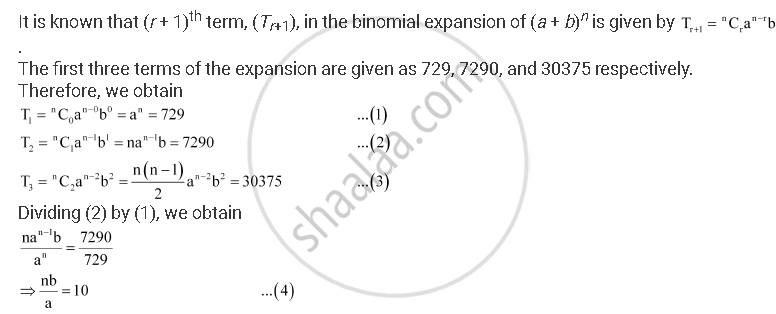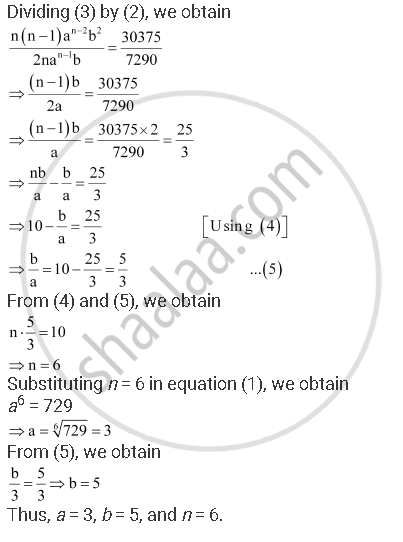CBSE (Arts) Class 11CBSE
Share
Notifications

View all notifications
Books Shortlist
Your shortlist is empty

# Find A, B and N in the Expansion of (A + B)N If the First Three Terms of the Expansion Are 729, 7290 and 30375, Respectively. - CBSE (Arts) Class 11 - Mathematics

Login
Create free account

Forgot password?
ConceptBinomial Theorem for Positive Integral Indices

#### Question

Find ab and n in the expansion of (a + b)n if the first three terms of the expansion are 729, 7290 and 30375, respectively.

#### SolutionIs there an error in this question or solution?

#### APPEARS IN

Solution Find A, B and N in the Expansion of (A + B)N If the First Three Terms of the Expansion Are 729, 7290 and 30375, Respectively. Concept: Binomial Theorem for Positive Integral Indices.
S